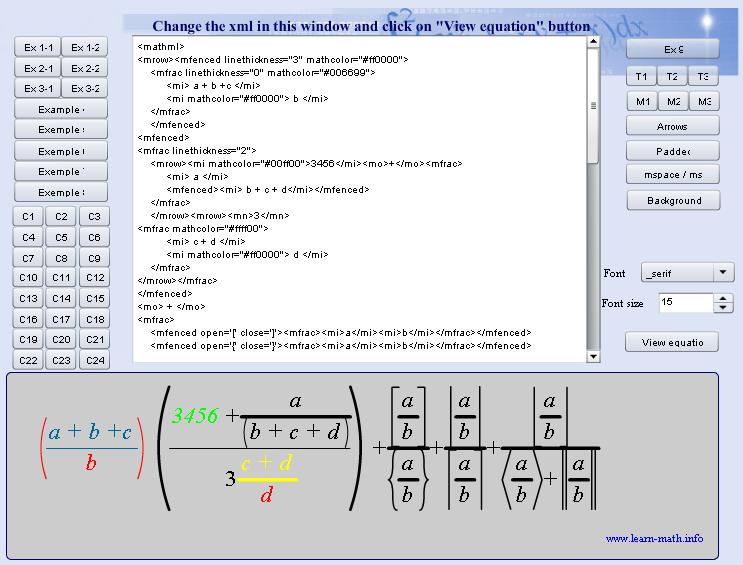# MathML Formula

4 收藏

MathML Formula 正在参加 2020 年度 OSC 中国开源项目评选，请投票支持！
MathML Formula 在 2020 年度 OSC 中国开源项目评选 中已获得 {{ projectVoteCount }} 票，请投票支持！

MathMLFormula 是一个 Flash 开发的组件用于在 Web 上显示 MathML 公式。### MathML Formula 的相关资讯### MathML Formula 的相关博客### MathML Formula 的相关问答### 评论 (0)0 评论
4 收藏